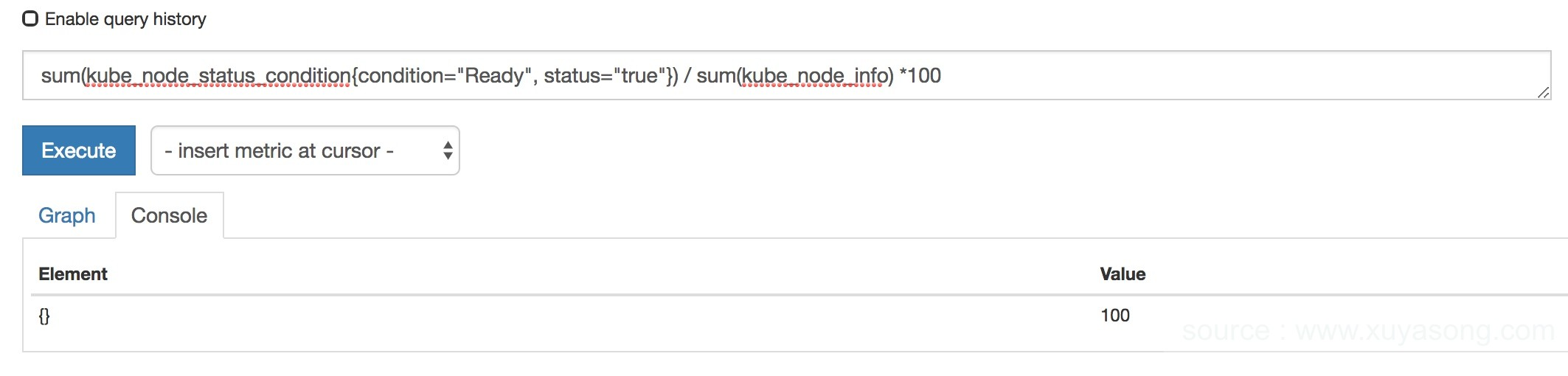### 一. 概述

Prometheus除了存储数据外，还提供了一种强大的功能表达式语言 PromQL，允许用户实时选择和汇聚时间序列数据。### Metric类型

Prometheus会将所有采集到的样本数据以时间序列（time-series）的方式保存在内存数据库TSDB中，并且定时保存到硬盘上。time-series是按照时间戳和值的序列顺序存放的，我们称之为向量(vector)。每条time-series通过指标名称(metrics name)和一组标签集(labelset)命名。

• 指标(metric)：metric name和描述当前样本特征的labelsets;
• 时间戳(timestamp)：一个精确到毫秒的时间戳;
• 样本值(value)： 一个folat64的浮点型数据表示当前样本的值。


node_cpu{app="node-exporter",cpu="cpu0",instance="192.168.0.4:9100",job="kubernetes-service-endpoints",kubernetes_name="node-exporter",kubernetes_namespace="kube-system",mode="guest"}	 459.71


Prometheus定义了4中不同的指标类型(metric type):

• Counter 计数器


rate(http_requests_total[5m])

• Gauge 仪表盘



• Histogram 直方图

Histogram 由 <basename>_bucket{le="<upper inclusive bound>"}，<basename>_bucket{le="+Inf"}, <basename>_sum，<basename>_count 组成，主要用于表示一段时间范围内对数据进行采样（通常是请求持续时间或响应大小），并能够对其指定区间以及总数进行统计，通常它采集的数据展示为直方图。


• Summary 摘要

Summary 和 Histogram 类似，由 <basename>{quantile="<φ>"}，<basename>_sum，<basename>_count 组成，主要用于表示一段时间内数据采样结果（通常是请求持续时间或响应大小），它直接存储了 quantile 数据，而不是根据统计区间计算出来的。

Histogram 需要通过 <basename>_bucket 计算 quantile, 而 Summary 直接存储了 quantile 的值。



### 基础查询

PromQL是Prometheus内置的数据查询语言，其提供对时间序列数据丰富的查询，聚合以及逻辑运算能力的支持。


http_requests_total{job="prometheus",group="canary"}




=：选择正好相等的字符串标签
!=：选择不相等的字符串标签
=~：选择匹配正则表达式的标签（或子标签）
!=：选择不匹配正则表达式的标签（或子标签）



• s - 秒
• m - 分钟
• h - 小时
• d - 天
• w - 周
• y - 年

### 偏移查询

rate(http_requests_total{} offset 1w)


http_requests_total offset 5m

http_requests_total{job="prometheus"}[5m]

sum(http_requests_total{method="GET"} offset 5m) // GOOD.

sum(http_requests_total{method="GET"}) offset 5m // INVALID.

### 操作符

Prometheus 的查询语言支持基本的逻辑运算和算术运算

##### 二元算术运算：
• 加法
• 减法
• 乘法
• / 除法
• % 模
• ^ 幂等

• 瞬时向量（Instant vector） - 一组时间序列，每个时间序列包含单个样本，它们共享相同的时间戳。也就是说，表达式的返回值中只会包含该时间序列中的最新的一个样本值。而相应的这样的表达式称之为瞬时向量表达式。
• 区间向量（Range vector） - 一组时间序列，每个时间序列包含一段时间范围内的样本数据。
• 标量（Scalar） - 一个浮点型的数据值。
• 字符串（String） - 一个简单的字符串值。

node_disk_bytes_written + node_disk_bytes_read

node_memory_free_bytes_total / (1024 * 1024)

##### 布尔运算
• == (相等)
• != (不相等)
• (大于)

• < (小于)
• = (大于等于)

• <= (小于等于)


http_requests_total > 10000 # 结果为 true 或 false
http_requests_total > bool 10000 # 结果为 1 或 0


• and (并且)
• or (或者)
• unless (排除)
##### 优先级


100 * (1 - avg (irate(node_cpu{mode='idle'}[5m])) by(job) )



1. ^
2. *, /, %
3. +, -
4. ==, !=, <=, <, >=, >
5. and, unless
6. or
##### 匹配模式（联合查询）

• 一对一（one-to-one）
• 多对一（many-to-one）或一对多（one-to-many）


method_code:http_errors:rate5m{method="get", code="500"}  24
method_code:http_errors:rate5m{method="get", code="404"}  30
method_code:http_errors:rate5m{method="put", code="501"}  3
method_code:http_errors:rate5m{method="post", code="500"} 6
method_code:http_errors:rate5m{method="post", code="404"} 21

method:http_requests:rate5m{method="get"}  600
method:http_requests:rate5m{method="del"}  34
method:http_requests:rate5m{method="post"} 120




method_code:http_errors:rate5m{code="500"} / ignoring(code) method:http_requests:rate5m



{method=“get”} 0.04 // 24 / 600 {method=“post”} 0.05 // 6 / 120


method_code:http_errors:rate5m / ignoring(code) group_left method:http_requests:rate5m



{method=“get”, code=“500”} 0.04 // 24 / 600 {method=“get”, code=“404”} 0.05 // 30 / 600 {method=“post”, code=“500”} 0.05 // 6 / 120 {method=“post”, code=“404”} 0.175 // 21 / 120





### 聚合操作

Prometheus 还提供了下列内置的聚合操作符，这些操作符作用域瞬时向量。可以将瞬时表达式返回的样本数据进行聚合，形成一个具有较少样本值的新的时间序列。

• sum (求和)
• min (最小值)
• max (最大值)
• avg (平均值)
• stddev (标准差)
• stdvar (标准差异)
• count (计数)
• count_values (对 value 进行计数)
• bottomk (样本值最小的 k 个元素)
• topk (样本值最大的k个元素)
• quantile (分布统计)

• without 用于从计算结果中移除列举的标签，而保留其它标签。
• by 则正好相反，结果向量中只保留列出的标签，其余标签则移除。

sum(http_requests_total) without (instance)

sum(http_requests_total) by (application, group)

sum(http_requests_total)

count_values 用于时间序列中每一个样本值出现的次数。count_values 会为每一个唯一的样本值输出一个时间序列，并且每一个时间序列包含一个额外的标签。


count_values("version", build_version)

{count="641"}   1
{count="3226"}  2
{count="644"}   4



**topk 和 bottomk **

topk(5, http_requests_total)



quantile 用于计算当前样本数据值的分布情况 quantile(φ, express) ，其中 0 ≤ φ ≤ 1



quantile(0.5, http_requests_total)

{}   656



### 内置函数

Prometheus 提供了其它大量的内置函数，可以对时序数据进行丰富的处理。如上文提到的irate


100 * (1 - avg (irate(node_cpu{mode='idle'}[5m])) by(job) )



rate(node_cpu[2m])

irate(node_cpu[2m])



irate同样用于计算区间向量的计算率，但是其反应出的是瞬时增长率。

irate函数是通过区间向量中最后两个两本数据来计算区间向量的增长速率。



irate函数相比于rate函数提供了更高的灵敏度，不过当需要分析长期趋势或者在告警规则中，irate的这种灵敏度反而容易造成干扰。

• abs()
• absent()
• ceil()
• changes()
• clamp_max()
• clamp_min()
• day_of_month()
• day_of_week()
• days_in_month()
• delta()
• deriv()
• exp()
• floor()
• histogram_quantile()
• holt_winters()
• hour()
• idelta()
• increase()
• irate()
• label_join()
• label_replace()
• ln()
• log2()
• log10()
• minute()
• month()
• predict_linear()
• rate()
• resets()
• round()
• scalar()
• sort()
• sort_desc()
• sqrt()
• time()
• timestamp()
• vector()
• year()
• _over_time()

### API访问

Prometheus当前稳定的HTTP API可以通过/api/v1访问

• 422 Unprocessable Entity 当表达式无法执行时。
• 503 Service Unavailiable 当请求超时或者被中断时。


{
"status": "success" | "error",
"data": <data>,

// 为error时，有如下报错信息
"errorType": "<string>",
"error": "<string>"
}



##### 瞬时数据查询

URL请求参数：

• query=：PromQL表达式。
• time=：用于指定用于计算PromQL的时间戳。可选参数，默认情况下使用当前系统时间。
• timeout=：超时设置。可选参数，默认情况下使用-query,timeout的全局设置。
$curl 'http://localhost:9090/api/v1/query?query=up&time=2015-07-01T20:10:51.781Z'  返回： { "status" : "success", "data" : { "resultType" : "vector", "result" : [ { "metric" : { "__name__" : "up", "job" : "prometheus", "instance" : "localhost:9090" }, "value": [ 1435781451.781, "1" ] }, { "metric" : { "__name__" : "up", "job" : "node", "instance" : "localhost:9100" }, "value" : [ 1435781451.781, "0" ] } ] } }  ##### 区间查询 URL请求参数： • query=: PromQL表达式。 • start=: 起始时间。 • end=: 结束时间。 • step=: 查询步长。 • timeout=: 超时设置。可选参数，默认情况下使用-query,timeout的全局设置。 $ curl 'http://localhost:9090/api/v1/query_range?query=up&start=2015-07-01T20:10:30.781Z&end=2015-07-01T20:11:00.781Z&step=15s'


{
"status" : "success",
"data" : {
"resultType" : "matrix",
"result" : [
{
"metric" : {
"__name__" : "up",
"job" : "prometheus",
"instance" : "localhost:9090"
},
"values" : [
[ 1435781430.781, "1" ],
[ 1435781445.781, "1" ],
[ 1435781460.781, "1" ]
]
},
{
"metric" : {
"__name__" : "up",
"job" : "node",
"instance" : "localhost:9091"
},
"values" : [
[ 1435781430.781, "0" ],
[ 1435781445.781, "0" ],
[ 1435781460.781, "1" ]
]
}
]
}
}